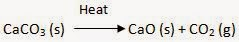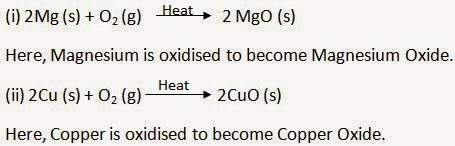## Breaking

CustomWritings.com - get an essay written for you by academic experts. Feel free to get writing assistance online.

## NCERT Solutions for Class 10 Science Chapter 1 – Chemical Reactions and Equations

In Text Questions

Page No: 6

1. Why should a magnesium ribbon be cleaned before it is burnt in air?

Magnesium is a very reactive metal. When stored, it reacts with oxygen to form a layer of magnesium oxide on its surface. This layer of magnesium oxide is quite stable and prevents further reaction of magnesium with oxygen. The magnesium ribbon is cleaned by sand paper for removing this layer so that the underlying metal can be exposed to air.

2. Write the balanced equation for the following chemical reactions.

(i) Hydrogen + Chlorine → Hydrogen chloride
(ii) Barium chloride + Aluminium sulphate → Barium sulphate + Aluminium chloride
(iii) Sodium + Water → Sodium hydroxide + Hydrogen

(i) H2 (g) + Cl2 (g) → 2HCl (g)

(ii) 3BaCl2 (s) + Al2(SO4)3 (s) → 3BaSO4 (s) + 2AlCl3 (s)

(iii) 2Na(s) + 2H2O (l) → 2NaOH (aq) + H2 (g)

3. Write a balanced chemical equation with state symbols for the following reactions.

(i) Solutions of barium chloride and sodium sulphate in water react to give insoluble barium sulphate and the solution of sodium chloride.
(ii) Sodium hydroxide solution (in water) reacts with hydrochloric acid solution (in water) to produce sodium chloride solution and water.

(i) BaCl2 (aq) + Na2SO4 (aq) → BaSO4 (s) + 2NaCl (aq)

(ii) NaOH (aq) + HCl (aq) → NaCL (aq) + H2O (l)

Page No: 10

1. A solution of a substance ‘X’ is used for white washing.
(i) Name the substance ‘X’ and write its formula.
(ii) Write the reaction of the substance ‘X’ named in (i) above with water.

(i) The substance ‘X’ is calcium oxide. Its chemical formula is CaO.

(ii) Calcium oxide reacts vigorously with water to form calcium hydroxide (slaked lime).
CaO (s)                                    + H2O (l) → Ca(OH)2 (aq)
Calcium Oxide (Quick Lime) + Water    → Calcium Hydroxide (Slaked Lime)

2. Why is the amount of gas collected in one of the test tubes in Activity 1.7 double of the amount collected in the other? Name this gas.

Water contains two parts of hydrogen and one part oxygen. Therefore, during the electrolysis of water the amount of hydrogen gas collected in one of the test tubes is double than that of the oxygen produced and collected in the other test tube.

Page No: 13

1. Why does the colour of copper sulphate solution change when an iron nail is dipped in it?

When an iron nail dipped in the copper sulphate solution than iron displaces copper from the copper sulphate because iron is more reactive than copper. Therefore the colour of the copper sulphate solution changes.

The reaction involved here is:
Fe (s) + CuSO4 (aq) → FeSO4 (aq) + Cu (s)

2. Give an example of a double displacement reaction other than the one given in Activity 1.10.

2KBr (aq) + BaI2 (aq) → 2KI (aq) + BaBr2 (aq)

3. Identify the substances that are oxidised and the substances that are reduced in the following reactions.
(i) 4Na (s) + O2 (g) → 2Na2O (s)
(ii) CuO (s) + H2 (g) → Cu (s) + H2O (l)

(i) Sodium (Na) is oxidised as it gains oxygen and oxygen gets reduced.

(ii) Copper oxide (CuO) is reduced to copper (Cu) while hydrogen (H2) gets oxidised to water (H2O).

Exercise
Page No: 14
1. Which of the statements about the reaction below are incorrect?
2PbO (s) + C (s) → 2Pb (s) + CO2 (g)
(b) Carbon dioxide is getting oxidised.
(c) Carbon is getting oxidised.
(d) Lead oxide is getting reduced.

(i) (a) and (b)
(ii) (a) and (c)
(iii) (a), (b) and (c)
(iv) all
► (i) (a) and (b)

2. Fe2O3 + 2Al → Al2O3 + 2Fe
The above reaction is an example of a
(a) combination reaction.
(b) double displacement reaction.
(c) decomposition reaction.
(d) displacement reaction.
► (d) displacement reaction.
Page No: 15

3. What happens when dilute hydrochloric acid is added to iron filings? Tick the correct answer.
(a) Hydrogen gas and iron chloride are produced.
(b) Chlorine gas and iron hydroxide are produced.
(c) No reaction takes place.
(d) Iron salt and water are produced. ► (a) Hydrogen gas and iron chloride are produced.

4. What is a balanced chemical equation? Why should chemical equations be balanced?

A reaction which has an equal number of atoms of all the elements on both sides of the chemical equation is called a balanced chemical equation.Chemical reaction should be balanced to follow law of conservation of mass.

5. Translate the following statements into chemical equations and then balance them.

(a) Hydrogen gas combines with nitrogen to form ammonia.
(b) Hydrogen sulphide gas burns in air to give water and sulphur dioxide.
(c) Barium chloride reacts with aluminium sulphate to give aluminium chloride and a precipitate of barium sulphate.

(d) Potassium metal reacts with water to give potassium hydroxide and hydrogen gas.

(a) 3H2 (g) + N2 (g) → 2NH3 (g)

(b) 2H2S (g) + 3O2 (g) → 2H2O (l) + 2SO2 (g)

(c) 3BaCl2 (aq) + Al2(SO4)3 (aq) → 2AlCl3 (aq) + 3BaSO4 (s)

(d) 2K (s) + 2H2O (l) → 2KOH (aq) + H2 (g)

6. Balance the following chemical equations.

(i) HNO3 + Ca(OH)2 → Ca(NO3)2 + H2O
(ii) NaOH + H2SO4 → Na2SO4 + H2O
(iii) NaCl + AgNO3 → AgCl + NaNO3
(iv) BaCl2 + H2SO4 → BaSO4 + HCl

(i) 2HNO3 + Ca(OH)2 → Ca(NO3)2 + 2H2O
(ii) 2NaOH + H2SO4 → Na2SO4 + 2H2O
(iii) NaCl + AgNO3 → AgCl + NaNO3
(iv) BaCl2 + H2SO4 → BaSO4 + 2HCl

7. Write the balanced chemical equations for the following reactions.

(a) Calcium hydroxide + Carbon dioxide → Calcium carbonate + Water
(b) Zinc + Silver nitrate → Zinc nitrate + Silver
(c) Aluminium + Copper chloride → Aluminium chloride + Copper
(d) Barium chloride + Potassium sulphate → Barium sulphate + Potassium chloride

(a) Ca(OH)2 + CO2 → CaCO3 + H2O

(b) Zn + 2AgNO3 → Zn(NO3)2 + 2Ag

(c) 2Al + 3CuCl2 → 2AlCl3 + 3Cu

(d) BaCl2 + K2SO4 → BaSO4 + 2KCl

8. Write the balanced chemical equation for the following and identify the type of reaction in each case.

(a)Potassium bromide (aq) + Barium iodide (aq)­ → Potassium iodide (aq) + Barium bromide(s)
(b) Zinc carbonate (s) → Zinc oxide (s) + Carbon dioxide (g)
(c) Hydrogen (g) + Chlorine (g) → Hydrogen chloride (g)
(d) Magnesium (s) + Hydrochloric acid (aq) → Magnesium chloride (aq) + Hydrogen (g)

(a) 2KBr (aq) + BaI2 (aq) → 2KI (aq) + BaBr2 (s): Double displacement reaction

(b) ZnCO3 (s) →  ZnO (s) + CO2 (g): Decomposition reaction

(c) H2 (g) + Cl2 (g) → 2HCl (g): Combination reaction

(d) Mg (s) + 2HCl (aq) → MgCl2 (aq) + H2 (g) : Displacement Reaction

9. What does one mean by exothermic and endothermic reactions? Give examples.

Chemical reactions that release energy in the form of heat, light, or sound are called exothermic reactions.

Example: C (g) + O2 (g) → CO2 + Heat Energy

Reactions that absorb energy or require energy in order to proceed are called endothermic reactions.
Example:

10. Why is respiration considered an exothermic reaction? Explain.

Respiration is considered as an exothermic reaction because in respiration oxidation of glucose takes place which produces large amount of heat energy.
C6H12O6 (aq) + 6O2 (g) → 6CO2 (g) + 6H2O (l) + Energy

11. Why are decomposition reactions called the opposite of combination reactions? Write equations for these reactions.

Decomposition reactions are those in which a compound breaks down to form two or more substances. These reactions require a source of energy to proceed. Thus, they are the exact opposite of combination reactions in which two or more substances combine to give a new substance with the release of energy.For Example:
Decomposition Reaction:Combination Reaction:
CaO (s) + H2O (l) → Ca(OH)2 (aq)

Page No: 16

12. Write one equation each for decomposition reactions where energy is supplied in the form of heat, light or electricity.13. What is the difference between displacement and double displacement reactions? Write equations for these reactions.

In a displacement reaction, a more reactive element replaces a less reactive element from a compound.
For Example: CuSo4 (aq) + Zn (s) → ZnSO4 (aq) + Cu (s)
In a double displacement reaction, two atoms or a group of atoms switch places to form new compounds.

For Example: Na2SO4 (aq) + BaCl2 (aq) → BaSO4 (s) + 2NaCl (aq)

14. In the refining of silver, the recovery of silver from silver nitrate solution involved displacement by copper metal. Write down the reaction involved.

2AgNO3 (aq) + Cu (s) → Cu(NO3)2 (aq) + 2Ag (s)
Silver Nitrate + Copper → Copper Nitrate + Silver

15. What do you mean by a precipitation reaction? Explain by giving examples.

A reaction in which an insoluble solid (called precipitate) is formed is called a precipitation reaction.For Example:
Na2CO3 (aq) + CaCl2 (aq) → CaCO3 (s) + 2NaCl (aq)
Sodium Carbonate + Calcium Chloride → Calcium Carbonate + Sodium Chloride
In this reaction, calcium carbonate is obtained as a precipitate. Hence, it is a precipitation reaction.

16. Explain the following in terms of gain or loss of oxygen with two examples each.
(a) Oxidation
(b) Reduction

Oxidation Reaction: It is a chemical reaction in which gain of oxygen or loss of hydrogen takes place.Reduction Reaction: It is a chemical reaction in which loss of oxygen or gain of hydrogen takes place.17. A shiny brown-coloured element ‘X’ on heating in air becomes black in colour. Name the element ‘X’ and the black coloured compound formed.

‘X’ is copper (Cu) and the black-coloured compound formed is copper oxide (CuO). The equation of the reaction involved on heating copper is given below.18. Why do we apply paint on iron articles?

Iron articles are painted because it prevents them from rusting. When painted, the contact of iron articles from moisture and air is cut off. Hence, rusting is prevented.

19. Oil and fat containing food items are flushed with nitrogen. Why?

Oil and fat containing food items flushed with nitrogen because nitrogen acts as an antioxidant and it prevents them from being oxidised.

20. Explain the following terms with one example each.
(a) Corrosion
(b) Rancidity